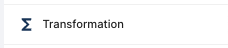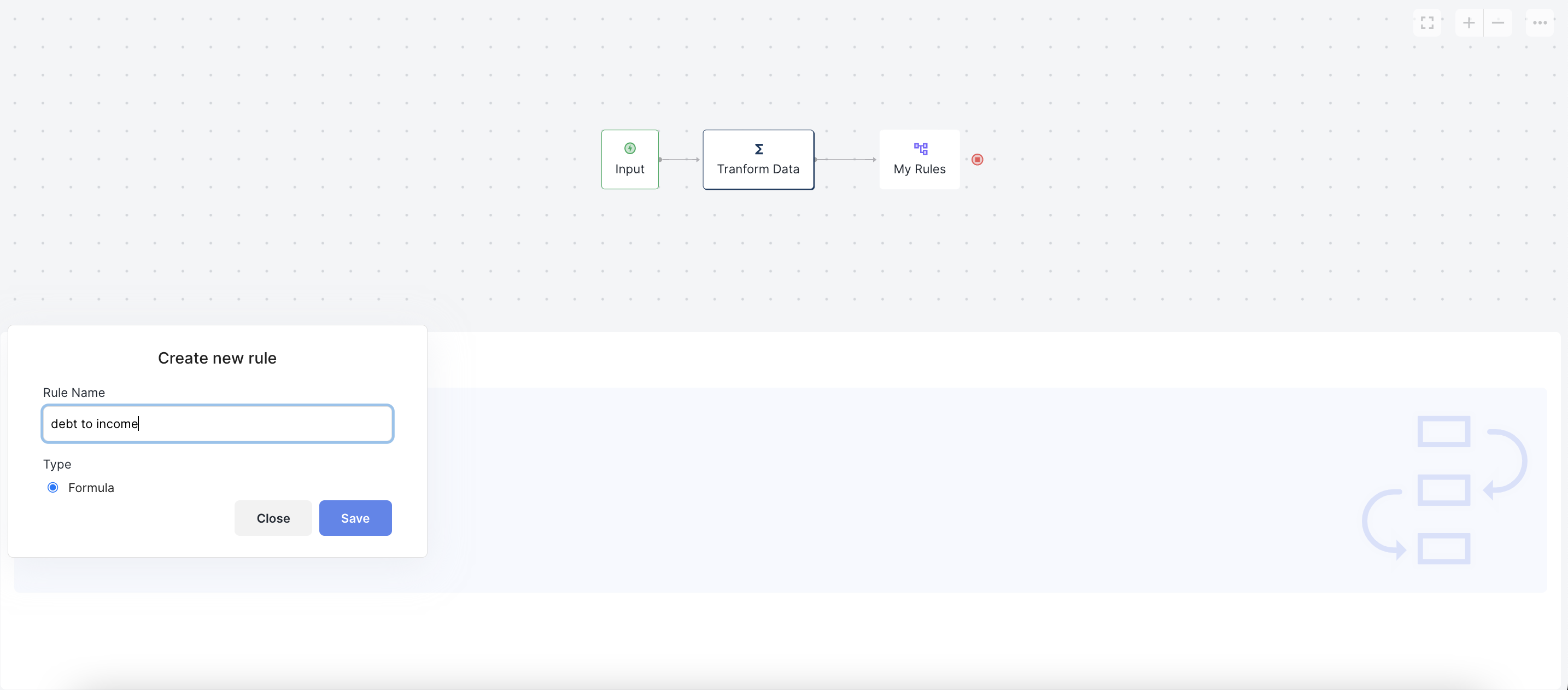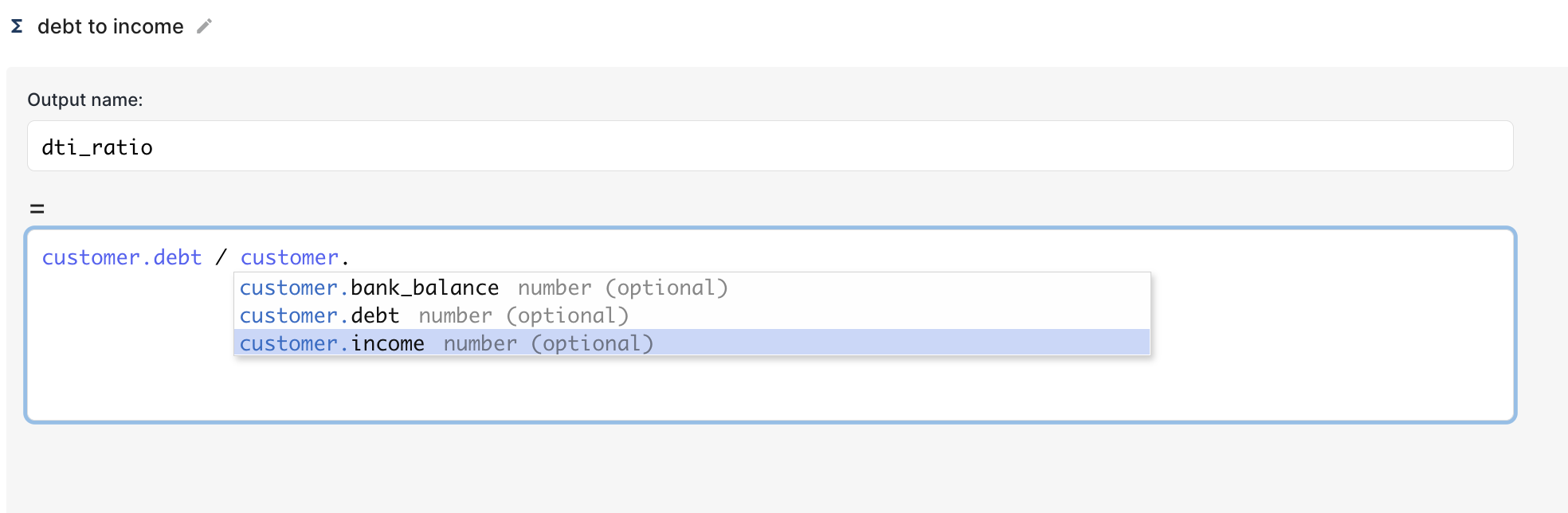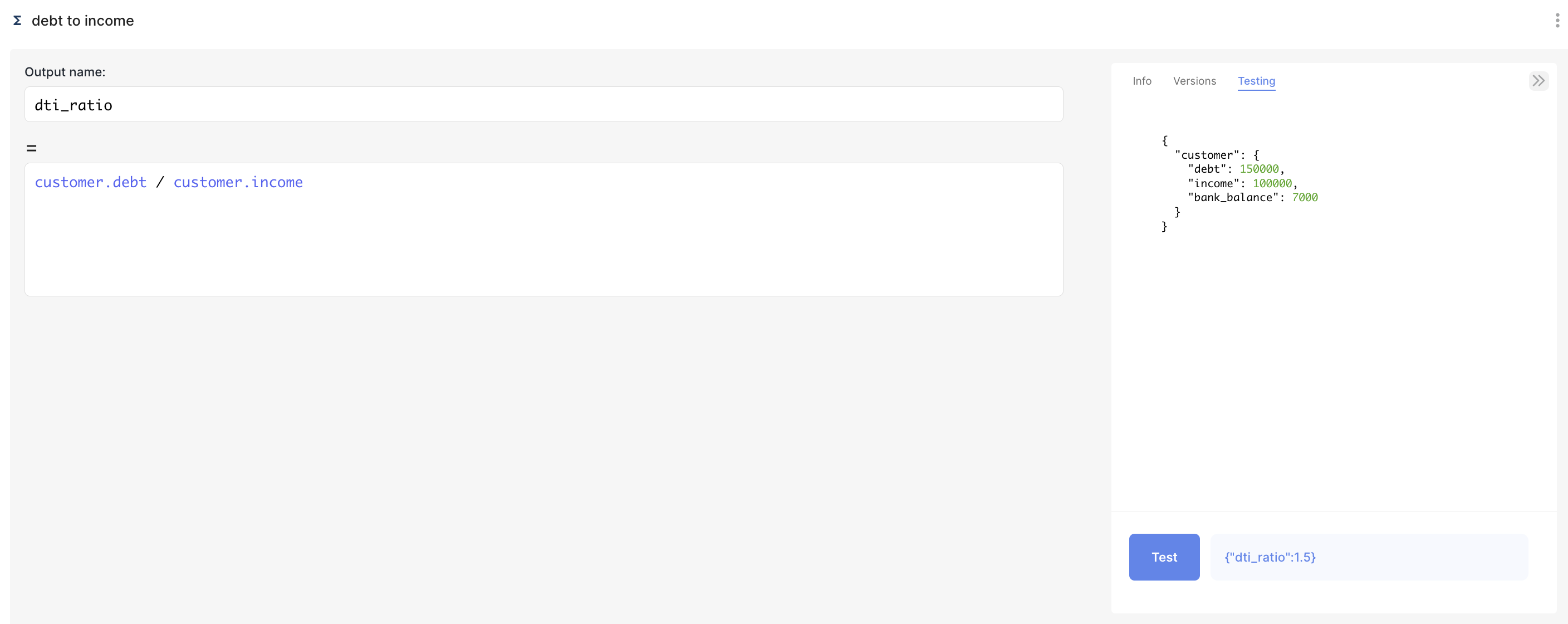# Transformation Blocks

Transformation Blocks are used to run a special type of rule called a Formula, used to create new Custom Variables by transforming data fields from other parts of your workflow.These can be used to calculate helper variables from your various data inputs, to use in your rules. A classic example would be financial ratios in a credit workflow, e.g "debt to income ratio"

As an example, lets use a workflow where we have both `customer.debt` and `customer.income` as input fields and we want to use `dti_ratio` in a few different rules, without writing out a formula every time we want to use it.

Instead, we can add a new Transform block to the graph, and call it 'Transform Data'. Then create a new Formula Rule like the example below.Let's create a new Formula rule, and create a new Custom Variable called `dti_ratio`We can then set this equal to our customer's debt/income, to create a new value for use in our rules. We can use any standard mathematical operators, as well as any of the Inline Functions

Once you've saved, the new custom variable `dti_ratio` will be available under autocomplete in all subsequent rules in your Workflow.

### Testing Formula Rules

You can test your Formula rules by clicking on the testing panel on the right of the Rule Editor. You can edit the sample input fields, and then click "Test" at the bottom to make sure your Formula returns what you'd expect. Click Save when you're done